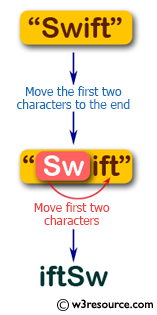﻿ Swift String Programming Exercise: Move the first two characters of a given string to the end - w3resource# Swift String Exercises: Move the first two characters of a given string to the end

## Swift String Programming: Exercise-9 with Solution

Write a Swift program to move the first two characters of a given string to the end. The given string length must be at least 2.

Pictorial Presentation:Sample Solution:

Swift Code:

``````func first_to_last(_ str1: String) -> String {
var chars = str1.characters
let first_char = chars.removeFirst()
let second_char = chars.removeFirst()
chars.append(first_char)
chars.append(second_char)

return String(chars)
}
print(first_to_last("Swift"))
print(first_to_last("Python"))
```
```

Sample Output:

```
iftSw
thonPy
```

Swift Programming Code Editor:

Improve this sample solution and post your code through Disqus

What is the difficulty level of this exercise?

﻿